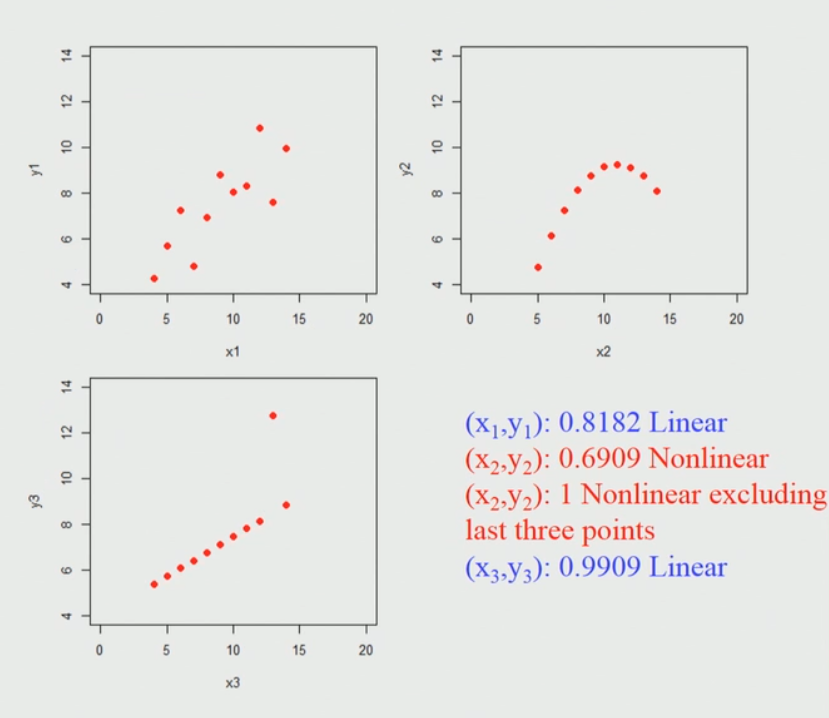Related Articles
Spearman’s Rank Correlation
• Last Updated : 18 Aug, 2020

What is correlation test?
The strength of the association between two variables is known as the correlation test. For instance, if we are interested to know whether there is a relationship between the heights of fathers and sons, a correlation coefficient can be calculated to answer this question.

Methods for correlation analysis:
There are mainly two types of correlation:

• Parametric Correlation – Pearson correlation(r) : It measures a linear dependence between two variables (x and y) is known as a parametric correlation test because it depends on the distribution of the data.
• Non-Parametric Correlation – Kendall(tau) and Spearman(rho): They are rank-based correlation coefficients, are known as non-parametric correlation.

Spearman Correlation formula:where,
rs = Spearman Correlation coefficient
di = the difference in the ranks given to the two variables values for each item of the data,
n = total number of observation

Example: In the Spearman’s rank correlation what we do is convert the data even if it is real value data to what we call ranks. Let’s consider taking 10 different data points in variable X1 and Y1. And find out their respective ranks. Then find out the square of the difference in the ranks given to the two variables values for each item of the data.

Number 1 2 3 4 5 6 7 8 9 10
X1 7 6 4 5 8 7 10 3 9 2
Y1 5 4 5 6 10 7 9 2 8 1
Rank X1 6.5 5 3 4 8 6.5 10 2 9 1
Rank Y1 4.5 3 4.5 6 10 7 9 2 8 1
d2 4 4 2.25 4 4 0.25 1 0 1 0

Step 1: Finding Rank-

• Rank X1: So, what we have done is looked at all the individual values of X1 and assigned a rank to it. For example, the lowest value, in this case, is 2 and it is given a rank 1 the next highest value is 3 that is given a rank 2 and so on. So, we are ranked all of these points. Notice that the sixth and the first value both are tied. So, they get the rank of 6.5(the midway the half of it) because there is a tie. Similarly, if there are more than 2 values that are tied we take all these ranks and average them by the number of data points that have equal values, and correspondingly you have to give the rank.
• Rank Y1: Similarly, you can give rank to Y1 data points in the same manner.

Step 2: Calculate d2
Once you have got the rank you compute the difference in the ranks. So, in this case, the difference in the rank for the first data point is 2 and we square it, similarly, we take the difference in the second data point in the ranks between Xi and Yi which is 2 and square it and we get 4. So, like this, we make the difference in the ranks and by squaring it we get the final what we call the d squared values. We sum overall values and then we compute the Spearman coefficient by using this value in the above formula.

By putting the value of the overall sum of d2 and n value

rho/rs = 1 - ((6 x 20.5) / 990)
= 1 - (123 / 990)
= 1 - 0.1242
= 0.88


Properties:

• rs takes a value between -1(negative association) and 1(positive association).
• rs = 0 means no association.
• It can be used when association is non linear.
• It can be applied for ordinal variables.

Spearman Correlation for Anscombe’s Data:
Anscombe’s data also known as Anscombe’s quartet comprises of four datasets that have nearly identical simple statistical properties, yet appear very different when graphed. Each dataset consists of eleven (x, y) points. They were constructed in 1973 by the statistician Francis Anscombe to demonstrate both the importance of graphing data before analyzing it and the effect of outliers on statistical properties.